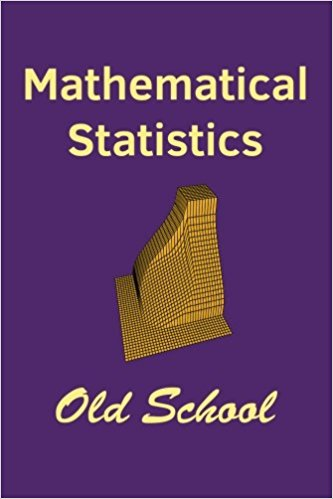# Mathematical Statistics: Old SchoolMathematical Statistics: Old School covers three main areas: The mathematics needed as a basis for work in statistics; the mathematical methods for carrying out statistical inference; and the theoretical approaches for analyzing the efficacy of various procedures. The author, John Marden, developed this material over the last thirty years teaching various configurations of mathematical statistics and decision theory courses in the Department of Statistics, University of Illinois at Urbana-Champaign. It is intended as a graduate-level text.

Topics include

• Distribution theory: distribution functions, densities, moment generating functions, transformations, the multivariate normal distribution, Bayes theorem, order statistics, convergence in probability and distribution, and the Δ-method.
• Statistical Inference: estimation and hypothesis testing, as well as confidence intervals and model selection, from both a frequentist and Bayesian perspective; exponential family and linear regression models, likelihood methods, and bootstrap and randomization techniques.
• Statistical decision theory: uniformly minimum variance estimators, the Cramér-Rao lower bound, uniformly most powerful tests, invariance, admissibility, and minimaxity.The pdf version of the book. (Free!) Some corrections.

Buy a hard copy: Only \$29.95!

Many of the data sets can be found as R objects in the file mathstat.R. Load them directly into R using the command

``` source("http://www.stat.istics.net/MathStat/mathstat.R") ```

The data sets, and where they are first mentioned:
• livepref: Ranks of where people prefer to live, from Section 3.2.1.
• smokingDeath: Relationship of age, smoking status, and being alive, from Exercise 6.8.2.
• caffeine: Effect of caffeine on short-term memory, from Exercise 11.7.10.
• shoes: # of pair of shoes owned vs. shoes size, from Section 11.5.1.
• hurricanes1: Data on hurricane damage vs. name on a masculine/feminine scale, from Section 12.5.2.
• greek: Relationship between being in a fraternity/sorority and # of hours partying, from Section 14.8.3.
• snoring: Snoring vs. heart disease, from Exercise 14.9.7.
• nl2015: Pairwise results from the National League (baseball) in 2015, from Exercise 14.9.9.
• politics: Intent to vote vs. education and political interest, from Exercise 16.7.7.
• draft69, draft69m, draft70, draft70m: Draft lottery data for 1969 and 1970, by day and by month, from Section 17.3 and Exercise 18.5.13.
• treadwear: Comparison on two methods for measuring tread wear on tires, from Section 17.4.1.
• aca: Comparison of attitudes toward the Affordable Care Act, 'ACA' vs. 'Obamacare,' from Exercise 17.6.4.
• examscores: Relationship of hourly and final exam scores in a class, from Exercise 17.6.8.
• barley: Student's (W. S. Gosset) data on yields of barley based on seed treatment, from Exercise 18.5.2.
The following data sets are in the Data Program as well: Caffeine, Shoe numbers and sizes, Hurricanes, 'Being Greek,' the draft data, and 'The Grades' (exam scores).

• Home: Stat.Istics.Net — Bringing statistics into the 20th century
• Data Program: Analyze data — Histograms, scatter plots, multiple regression, chi-square tests of independence, logistic regression.
• Box models: Randomly draws tickets from a box, to see the law of averages and the central limit theorem.
• Guessing Correlations: Match correlations with scatter plots.
• Monty Hall: Win a new car!